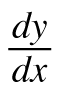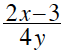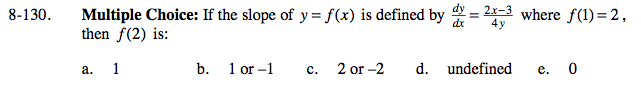### Home > CALC > Chapter 8 > Lesson 8.3.3 > Problem8-130

8-130.
1. Multiple Choice: If the slope of y = f(x) is defined by=where f(1) = 2, then f(2) is: Homework Help ✎

1. 1

2. 1 or −1

3. 2 or −2

4. undefined

5. 0Implicitly integrate to find the function f(x), so that you can evaluate f(2). Knowing that f(1) = 2 will help you find the value of C, the constant of integration.